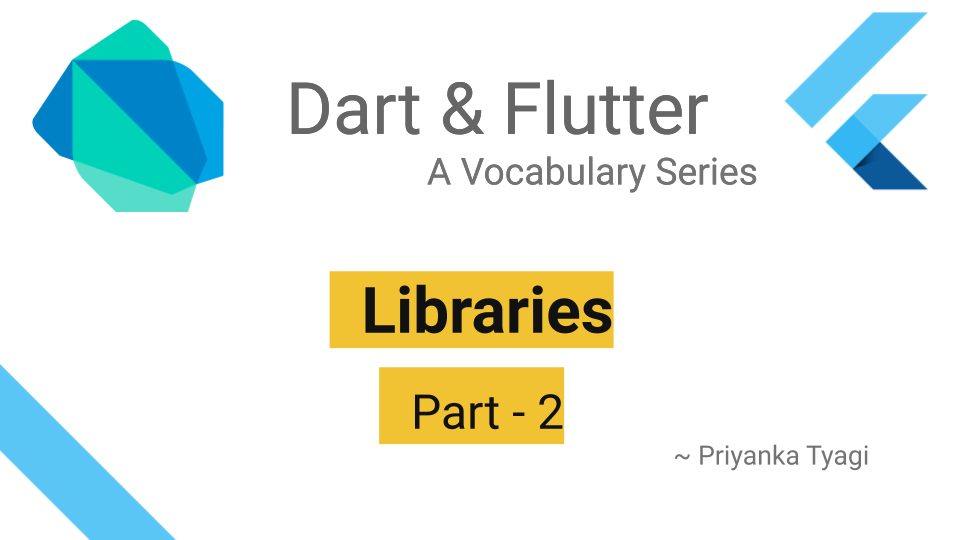# Dart Libraries (Part2)

Posted on May 10, 2020 in Dart# Introduction

This article covers `part`, `library`, and `export` directives. Checkout the Part-1 to learn few ways libraries are used in Dart. In addition to introducing above directives, we'll also be learning to restrict the visibility of a library's APIs.

# Revisiting Setup

Let's revisit the sample libraries `lib1` and `lib2`created in Part-1 for demonstration.

# `lib1`

This library has APIs to calculate sum and difference of two integers.

• Addition API: The `int addition(int a, int b)` calculates sum of two given integers and returns an integer.

• Subtraction API: The `int subtraction(int a, int b)` calculates difference of two given integers and returns an integer.

• `int _add(int a, int b)`: It is an internal function/method that does perform the real addition. This method is used by addition api. Identifiers with underscore (_) prefix are only available inside library.

SourceCode: Please refer to `lib1.dart`

``````  int addition(int a, int b) => _add(a, b);

int subtraction(int a, int b) => a - b;

int _add(int a, int b) => a + b;
``````

# `lib2`

This library has APIs to check if the given number is even or odd.

• Even number API (`bool isNumberOdd(int num)`): This api take a number and returns true if its even or vice versa.
• Odd number API (`bool isNumberOdd(int num)`): This api take a number and returns true if its odd or vice versa.

SourceCode: Please refer to `lib2.dart`

``````  bool isNumberOdd(int num) => num.isOdd;

bool isNumberEven(int num) => num.isEven;
``````

# Privacy (Restricting Access)

It's possible to restrict variables'/APIs visibility in Dart libraries. Identifiers with underscore (_) prefix are only available inside library.

Let's import `lib1.dart` to use `addition(..)` method. Please note that trying to call `_add(...)` method will show a compile time error since it's private and is available to `lib1.dart` only.

The `lib_main.dart`:

SourceCode: Please refer to `lib_main.dart`

``````import 'lib1.dart';

void main() {
int num1 = 5;
int num2 = 2;

print("Sum of \$num1 and \$num2 is \$sum");

//Compile-time error because _add() function is private
}
``````

Output:

``````Sum of 5 and 2 is 7
``````

# Using `part` directive

The use of `part` directive is most common in auto-generated code. Traditionally, `part` directive is a glue between two tightly coupled libraries.

Note: Related code is available in its own folder `libraries/maths_part`.

For example, lets that assume `lib1.dart` and `lib2.dart` are two huge libraries that were spilt into two. In normal circumstances, I would add both libraries in `import` statements at the top of `maths_part/part_main.dart` in order to access APIs from both libraries. In such scenarios, `part` directive could come handy to avoid multiple import statements.

The `lib1.dart` file: The `part` directive at the top mentions that all `lib2` belongs to `lib1`, and bring-in all `lib2`'s apis into `lib1`. Only importing `lib1.dart` will give access to both libraries' apis.

SourceCode: Please refer to `lib1.dart`

``````//importing lib1 brings-in all apis from lib2 as well
part 'lib2.dart';

int subtraction(int a, int b) => a - b;

int _add(int a, int b) => a + b;
``````

The `lib2.dart` file: This library is marked as part of `lib1.dart` by using `part of` directive as `part of 'lib1.dart';`.

SourceCode: Please refer to `lib2.dart`

``````part of 'lib1.dart';

bool isNumberOdd(int num) => num.isOdd;

bool isNumberEven(int num) => num.isEven;
``````

The `part_main.dart` file: Importing `lib1.dart` gives access to all apis from both `lib1` & `lib2`. `part_main.dart`

``````import 'lib1.dart';

void main() {
int num1 = 5;
int num2 = 2;

//API from lib1.dart
print("Sum of \$num1 and \$num2 is \$sum");

//API from lib2.dart
print("is number even? \${isNumberEven(num2)}");
}
``````

Output:

``````Sum of 5 and 2 is 7
is number even? true
``````

# `library` directive

The `library` directive is used to auto-generate documentation for library using `dartdoc` tool.

SourceCode: Please refer to `math_lib1` for this example.

# Generating documentation

1. Add `library` directive at the top of `lib1.dart`:
``````library math_lib1;
``````
1. Create a directory `math_lib1`, and copy `lib1.dart` under it.

2. Add `pubspec.yaml` under `math_lib1` directory:

``````name: math_lib1
description: Math operations library
version: 0.0.1
``````
1. Use `dartdoc` tool to create documentation:
``````  \$ cd math_lib1

# Generates documentation
\$ dartdoc

# Documentation available at `http://localhost:8080/`
\$ dhttpd --path doc/api
``````

# Using `export` directive

This directive is used to export only public apis. In other words, it makes apis publicly accessible.

SourceCode: Please refer to `math_export` for this example.

The `lib1.dart` and `lib2.dart` files are available under `lib/src/` folder. The files under `lib/src/` directory are hidden. The `lib/maths_export.dart` file use `export` directive to make `lib1.dart` public.

The `lib/maths_export.dart`:

``````export 'src/lib1.dart';
``````

An external file `lib_export.dart` imports `maths_export.dart` like below:

The `lib_export.dart`:

``````import 'math_export/lib/maths_export.dart';

void main() {
int num1 = 5;
int num2 = 2;

print("Sum of \$num1 and \$num2 is \$sum");

//Compile-time error because lib2.dart is not exported
//print("is number even? \${isNumberEven(num2)}");
}
``````

Output:

``````Sum of 5 and 2 is 7
``````

# Summary

In this article, we saw how can `export`, `library`, and `part` directives are used in Dart. We touched on generating documentation from Dart libraries. We also saw how to keep apis private in libraries.

That's it for this article. Check out the Dart Vocabulary Series for other Dart stuff.

# Source Code

Please checkout the source code at Github here

Happy Darting :)